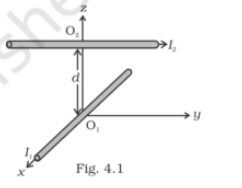# Two long wires carrying current I1 and I2 are arranged

Question:

Two long wires carrying current I1 and I2 are arranged as shown in the figure. The one carrying I1 is along is the x-axis. The other carrying current I2 is along a line parallel to the y-axis given by x = 0 and z = d. Find the force exerted at O2 because of the wire along the x-axis.Solution:

The magnetic field B on a current-carrying conductor is given as F = I(L×B) = ILB sinθ

O2 and I1 are parallel to the y-axis and are in the direction of –Y

I2 is parallel to the y-axis and is along Y-axis therefore, the angle between I2 and B1 is zero. The magnetic force F2 is given as F2 = B1I2L1 sin 0o = 0

Therefore, the force on O2 has current I1 zero.